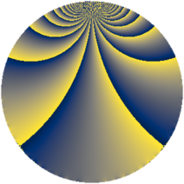# Properties

 Label 3724.1.bvLevel $3724$ Weight $1$ Character orbit 3724.bv Rep. character $\chi_{3724}(113,\cdot)$ Character field $\Q(\zeta_{14})$ Dimension $12$ Newform subspaces $1$ Sturm bound $560$ Trace bound $0$

# Related objects

## Defining parameters

 Level: $$N$$ $$=$$ $$3724 = 2^{2} \cdot 7^{2} \cdot 19$$ Weight: $$k$$ $$=$$ $$1$$ Character orbit: $$[\chi]$$ $$=$$ 3724.bv (of order $$14$$ and degree $$6$$) Character conductor: $$\operatorname{cond}(\chi)$$ $$=$$ $$931$$ Character field: $$\Q(\zeta_{14})$$ Newform subspaces: $$1$$ Sturm bound: $$560$$ Trace bound: $$0$$

## Dimensions

The following table gives the dimensions of various subspaces of $$M_{1}(3724, [\chi])$$.

Total New Old
Modular forms 66 12 54
Cusp forms 30 12 18
Eisenstein series 36 0 36

The following table gives the dimensions of subspaces with specified projective image type.

$$D_n$$ $$A_4$$ $$S_4$$ $$A_5$$
Dimension 12 0 0 0

## Trace form

 $$12q + 2q^{5} + q^{7} - 2q^{9} + O(q^{10})$$ $$12q + 2q^{5} + q^{7} - 2q^{9} - 5q^{11} - 5q^{17} + 12q^{19} - 4q^{23} - q^{35} + 2q^{43} - 5q^{45} + 2q^{47} + q^{49} - 9q^{55} + 2q^{61} + q^{63} + 2q^{73} - q^{77} - 2q^{81} - 4q^{83} - 2q^{85} + 2q^{95} + 2q^{99} + O(q^{100})$$

## Decomposition of $$S_{1}^{\mathrm{new}}(3724, [\chi])$$ into newform subspaces

Label Dim. $$A$$ Field Image CM RM Traces $q$-expansion
$$a_2$$ $$a_3$$ $$a_5$$ $$a_7$$
3724.1.bv.a $$12$$ $$1.859$$ $$\Q(\zeta_{21})$$ $$D_{21}$$ $$\Q(\sqrt{-19})$$ None $$0$$ $$0$$ $$2$$ $$1$$ $$q+(-\zeta_{42}-\zeta_{42}^{5})q^{5}-\zeta_{42}^{17}q^{7}+\cdots$$

## Decomposition of $$S_{1}^{\mathrm{old}}(3724, [\chi])$$ into lower level spaces

$$S_{1}^{\mathrm{old}}(3724, [\chi]) \cong$$ $$S_{1}^{\mathrm{new}}(931, [\chi])$$$$^{\oplus 3}$$